# 一个有关当前时间的sql排序实现

/ 0评 / 0

“在用户参与的一个活动列表中，获取当前时间中刚刚开始了(也就是刚才开始的，不是最先开始)的一条活动；如果当前时间没有活动开始，则获取将要开始的一条活动记录”且必须在一次数据库查询操作中完成。

id，starttime，endtime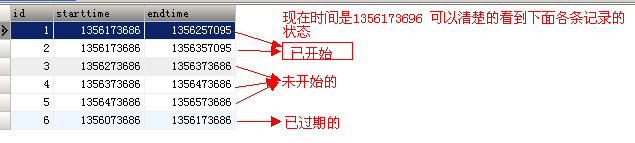mysql中的条件语句分支，常用的就是if ,case when 语句。

[sql]

if (SELECT * FROM `t_time` where starttime < UNIX_TIMESTAMP() and endtime >UNIX_TIMESTAMP())
then ( SELECT * FROM `t_time` where starttime < UNIX_TIMESTAMP() and endtime >UNIX_TIMESTAMP() order by starttime desc )
else if(SELECT * FROM t_time WHERE starttime > UNIX_TIMESTAMP() and endtime > UNIX_TIMESTAMP())
then ( SELECT * FROM `t_time` where starttime > UNIX_TIMESTAMP() and endtime >UNIX_TIMESTAMP() order by starttime asc )
end if;

[/sql]

Err] 1064 - You have an error in your SQL syntax;

[sql]

select * from t_time where endtime > UNIX_TIMESTAMP()
and (case when starttime < UNIX_TIMESTAMP() then starttime < UNIX_TIMESTAMP() else starttime > UNIX_TIMESTAMP() end) order by starttime desc;
[/sql]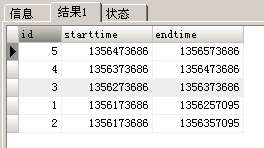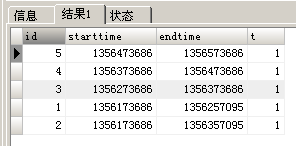IF(expr1,expr2,expr3)

if()函数可以返回数值或者是字符

[sql]

SELECT *,if(starttime < UNIX_TIMESTAMP(), 'starttime < UNIX_TIMESTAMP()', 'starttime > UNIX_TIMESTAMP()') as t
FROM `t_time` where endtime > UNIX_TIMESTAMP() and t order by starttime desc;

[/sql]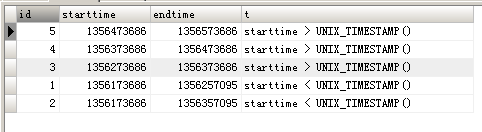if()函数确实是执行了，但是返回的字符 别名为t，sql语句还是不认识。这个没搞明白。

[sql]

SELECT *,if( starttime < UNIX_TIMESTAMP(), starttime - UNIX_TIMESTAMP(), (starttime - UNIX_TIMESTAMP()) + 2000000) as t
FROM `t_time` where endtime > UNIX_TIMESTAMP() order by t asc;

[/sql]

starttime - UNIX_TIMESTAMP()这样得到了 “starttime距离现在最近的，也就是马上将要开始的starttime”记录列表；由于 “未开始记录的”starttime - UNIX_TIMESTAMP() 得到的值可能小于“已开始记录的”starttime - UNIX_TIMESTAMP() 的值，这种情况下排序就混乱了，所以要加上一个很大的值20000000避免这种情况，这样就能保证未开始记录的starttime永远大于已开始记录的 starttime值，这样未开始的值记录排在了已开始记录之后了。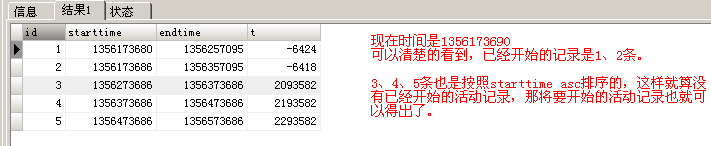( 图上的时间是假定的，就是为了说明已经开始的记录是哪几条。其实执行的时间也是算得出来的:-) )

starttime < UNIX_TIMESTAMP() ，那么starttime - UNIX_TIMESTAMP() 则是一定为负数的；starttime越大，得出的绝对值越小。反之starttime越小，得出的绝对值则越大，但加上负号，则是starttime越小的，得出的负值越小了，最后sql语句都是asc排序的。结果集里面已开始的记录也就是asc排序的。

[sql]

SELECT *,if( starttime < UNIX_TIMESTAMP(), UNIX_TIMESTAMP() - starttime, (starttime - UNIX_TIMESTAMP()) + 20000000) as t
FROM `t_time` where endtime > UNIX_TIMESTAMP() order by t asc;

[/sql]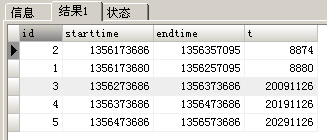• 默认
• 护眼
• 夜晚
• Serif
• Sans